# SAT Math Multiple Choice Question 905: Answer and Explanation

### Test Information

Question: 905

5.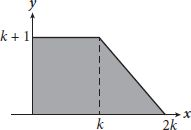Which of the following polynomial equations could be used to represent the area ofthe trapezoid shown in the figure?

• A.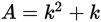• B.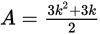• C.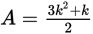• D.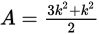Explanation:

B

Difficulty: Hard

Category: Passport to Advanced Math / Exponents

Strategic Advice: The area of a trapezoid is given by the formula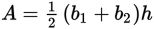where b1 and b2 represent the lengths of the bases and h represents the height of the trapezoid.

Getting to the Answer: Although this question asks about a polynomial equation, focus on what you're looking for-an equation that represents the area of the trapezoid. The polynomial part will take care of itself. Examine the figure along with the labels along the axes to determine the dimensions of the trapezoid (which happen to be variables instead of numbers). The height of the trapezoid is the vertical distance from the x-axis to the top base, which is given (labeled on the y-axis) as k + 1. The length of the top base is the distance from the y-axis to the dotted line inside the trapezoid, which is k. Finally, the length of the bottom base is the distance from the y-axis to the right-most point along the x-axis, which is 2k. Substitute these values into the area formula and simplify: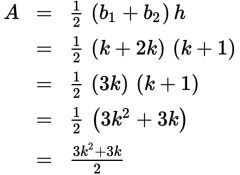This matches (B).